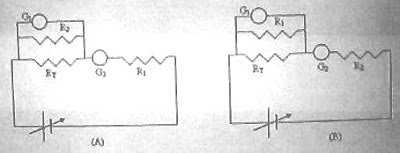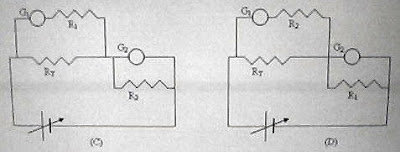## Friday, May 28, 2010

### Fluid Mechanics – Multiple Choice Practice Questions for AP Physics C and IIT-JEE

Questions served for AP Physics C exam. and IIT-JEE are generally not as simple as the questions served for other degree entrance exams. You require more practice with tougher questions to obtain higher scores in these exams. Here are a few multiple choice practice questions on fluid mechanics:

[There will be 5 options for AP Physics C exam., but 4 options only for IIT-JEE](1) A bowl has a small hole at the centre of its bottom. Water poured into the bowl drains through the hole and the height of water column at any instant t is H. The radius of the free surface of water is R (fig.) at the instant t. If the time rate of decrease of the height of water column is constant, how is H related to R?

(a) H α R1/2

(b) H α R

(c) H α R2

(d) H α R3

(e) H α R4

The time rate of decrease of the height of water column is dH/dt and we have

dH/dt = constant, as given in the question.

Since the velocity of the water flowing out (velocity of efflux) through the hole is √(2gH), the rate of flow is av = a√(2gH) where a is the area of the hole.

Therefore, we have

a√(2gH) = πR2(dH/dt)

Since a, g, π and dH/dt are costants, H α R4 [Option (e)].

(2) A jar of uniform area of cross section A has a hole of area a at its bottom. If there is water column of height H1 in the jar initially, what time is required for the height to become H2?

(a) (A/a) √(4/g) (√H1 H2)

(b) (A/a) √(2/g) (√H1 H2)

(c) (A/a) (4/g) (H1 H2)

(d) (A/a) √(1/g) (√H1 H2)

Rate of flow of water through the hole is av where v is the efflux velocity given by

v =√(2gx) where x is the height of water column.

If the height of water column changes by dx (fig.) in a time dt, we have

Adx (= avdt) = a√(2gx) dt

[The negative sign shows that x decreases].

Therefore, dt = (A/a)[1/√(2g)] (dx/x1/2)

Integrating between the limits x = H1 and x = H2 we obtain the required time t.

Thus t = (A/a)[1/√(2g)] H1H2 (x1/2)dx

Or, t = (A/a)[1/√(2g)] H2H1 (x1/2)dx

This gives t = (A/a) √(2/g) (√H1 H2)

(3) A sphere of wax (density 900 kgm–3) has a volume of 20 cm3. Iron nails are pierced into it so that it just gets submerged in water. If the volume of the iron nails is negligible compared to the volume of the sphere of wax, what is the mass of the iron nails in the sphere?

(a) 0.001 kg

(b) 0.002 kg

(c) 0.01 kg

(d) 0.02 kg

(e) 0.09 kg

Since the sphere of wax containing the iron nails gets just submerged in water, the mean density of the sphere must be equal to the density of water (1000 kgm–3).

Mass of wax in the sphere is 20×10–6×900 kg.

If m represents the mass of the nails, we have

(20×10–6×900 + m) /(20×10–6) = 1000

This gives m = 2×10–3 kg = 0.002 kg.

You will find a few more multiple choice questions (with solution) in this section here.

## Wednesday, May 19, 2010

### IIT-JEE 2010 – Multiple Choice Questions (Straight Objective Single Correct Answer Type) on Direct Current Circuits

Today we will discuss three multiple choice questions which appeared in IIT-JEE 2010 question paper:(1) Consider a thin square sheet of side L and thickness t, made of a material of resistivity ρ. The resistance between two opposite faces, shown by the shaded areas in the figure is

(A) directly proportional to L

(B) directly proportional to t

(C) independent of L

(D) independent of t

We have R = ρL/A where A is the cross section area which is equal to L t.

Therefore, R = ρL/Lt = ρ/t, which is independent of L [Option (C)].

(2) Incandescent bulbs are designed by keeping in mind that the resistance of their filament increases with increase in temperature. If at room temperature, 100 W, 60 W and 40 W bulbs have filament resistances R100, R60 and R40 respectively, the relation between these resistances is :

(A) 1/R100 = 1/R40 + 1/R60

(B) R100 = R40 + R60

(C) R100 > R60 > R40

(D) 1/R100 > 1/R60 > 1/R40

The power P dissipated in a resistance R is given by

P = V2/R where V is the voltage applied across the resistance.

This shows that the resistance of the filament is smaller if the wattage of the incandescent lamp is greater. In other words, the reciprocal of the filament resistance is greater if the wattage of the incandescent lamp is greater. This is true at all temperatures including room temperature. Therefore we have

1/R100 > 1/R60 > 1/R40

(3) To verify Ohm's law, a student is provided with a test resistor RT, a high resistance R1, a small resistance R2, two identical galvanometers G1 and G2, and a variable voltage ource V. The correct circuit to carry out the experiment isTo verify Ohm’s law, the current through RT is to be measured by connecting an ammeter in series with it and the voltage across RT is to be measured by connecting a voltmeter in parallel with it. One galvanometer is to be converted into an ammeter by connecting the small resistance R2 across it. The other galvanometer is to be converted into a voltmeter by connecting the high resistance R1 in series with it. So the correct circuit to carry out the experiment is the one shown in option (C).

You will find some multiple choice questions (with solution) on direct current circuits here.

## Thursday, May 06, 2010

### Alternating Current Circuits – Two Questions (MCQ) on Resonance

-->
"We have to do the best we can. This is our sacred human responsibility."
Albert Einstein
The phenomenon of resonance in alternating current circuits is an interesting one with many practical applications and questions involving resonance are often seen in entrance question papers. You may try searching for ‘resonance’ on this blog (making use of the search box provided on this page) to access all previous posts related to resonance.
Today we will discuss two multiple choice questions meant for high lighting the behaviour of AC circuits at resonance:
-->
(1) An alternating current source of negligible impedance has an emf 6 volt. It is connected in series with a series LCR circuit consisting of a 72 Ω resistor and an inductor-capacitor combination. The inductor offers a reactance of 120 Ω at the operating frequency and the power dissipated in the LCR circuit is 0.5 W. What is the reactance offered by the capacitor?
(a) 60 Ω
(b) 72 Ω
(c) 120 Ω
(d) 192 Ω
(e) Zero
It is always a good idea to check whether the circuit is at resonance (Question setters have a craze to set questions involving resonance).
Since the power is dissipated in the resistor alone, we have
V2/72 = 0.5 where V is the voltage across the resistor.
[Remember the power equation, P = V2/R]
We obtain V2 = 36 from which V = 6 volt.
The circuit is indeed at resonance since the entire supply voltage appears across the resistance.
At resonance the inductive reactance is equal to the capacitive reactance and hence the correct option is 120 Ω.
(2) In a series LCR circuit R = 200 Ω and the voltage and the frequency of the main supply are 220 V and 50 Hz respectively. On taking out the capacitance from the circuit the current lags behind the voltage by 30º. On taking out the inductor from the circuit the current leads the voltage by 30º. The power dissipated in the LCR circuit is
(1) 242 W
(2) 305 W
(3) 210 W
(4) 0 W
This question appeared in AIEEE 2010 question paper.
The phase lag of current produced by the inductance is equal in value to the phase lead of current produced by the capacitor. This means that in the LCR circuit connected to the 220 V, 50 Hz supply, there is no phase difference between the supply voltage and the current and the circuit behaves as a pure resistor. Indeed the circuit is at resonance and the entire supply voltage appears across the resistor.
Therefore, the power P dissipated in the LCR circuit is given by
P = V2/R = 2202/200 = 242 W.